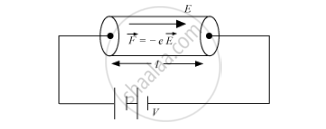Share

# Derive an expression for drift velocity of electrons in a conductor. Hence deduce Ohm's law. - CBSE (Science) Class 12 - Physics

ConceptDrift of Electrons and the Origin of Resistivity

#### Questions

Derive an expression for  drift velocity of free electrons.

Derive an expression for drift velocity of electrons in a conductor. Hence deduce Ohm's law.

#### Solution

(i) Free electrons are in continuous random motion. They undergo  change  in direction at each collision and the thermal velocities are randomly distributed in all directions.

∴ Average thermal velocity,u=(u_1+u_2+....+u_n)/n " is Zero".....(1)The electric field E exerts an electrostatic force ‘−Ee’.

Acceleration of each electron is,veca=(-evecE)/m " ......(2)"

Where,

m → Mass of an electron

→ Charge on an electron

Drift velocity,

vec(v_d)=(vec(v_1)+vec(v_2)+....+vec(v_n))/n

vec(v_d)=((vec(u_1)+vecat_1)+(vec(u_2)+vecat_2)+....+(vec(u_n)+vecat_n))/n

Where,

vecu_1,vecu_2-> Thermal velocities of the electrons

vecatau_1,vecatau_2-> Velocity acquired by electrons

τ1, τ2 → Time elapsed after the collision

vec(v_d)=((vec(u_1)+vec(u_2)+...+vecu_n))/n+(veca(vec(t_1)+vec(t_2)+...vec(t_n)))/n

Since (vec(u_1)+vec(u_2)+....vec(u_n))/n=0

∴ vτ

Where,t=(t_1+t_2+t_3....t_n)/n " is the average time elapsed"

Substituting for from equation (2),

vec(v_d)=(-evecE)/mt " ...(4)"

As, E=V/l
From (4) we can write

v_d=(eV)/(ml)τ
Also,
I=An""ev_d
Therefore,
I=An""e((eV)/(ml)τ)=(An""e^2τ)/(ml) V

or V/I=(ml)/(An""e^2τ)=R .... (5)

As we can see all the parameter on the R.H.S of the equation 5 are constant given temperature. And it is known as Resistance of the electric conductor.

Is there an error in this question or solution?

#### Video TutorialsVIEW ALL 

Solution Derive an expression for drift velocity of electrons in a conductor. Hence deduce Ohm's law. Concept: Drift of Electrons and the Origin of Resistivity.
S